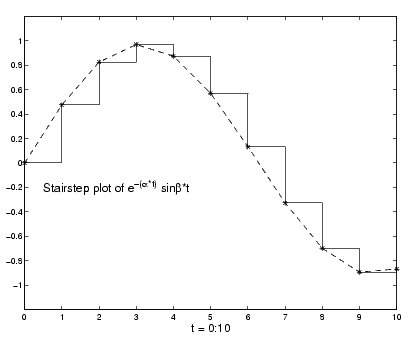GraphicsStairstep Plots

Stairstep plots display data as the leading edges of a constant interval (i.e., zero-order hold state). This type of plot holds the data at a constant y-value for all values between x(i) and x(i+1), where i is the index into the x data. This type of plot is useful for drawing time-history plots of digitally sampled data systems.

Example -- Stairstep Plot of a Function

Define a function f that varies over time,

• alpha = 0.01;
beta = 0.5;
t = 0:10;
f = exp(-alpha*t).*sin(beta*t);


Use stairs to display the function as a stairstep plot and a linearly interpolated function.

• stairs(t,f)
hold on
plot(t,f,'--*')
hold offAnnotate the graph and set the axes limits.

• label = 'Stairstep plot of e^{-(\alpha*t)} sin\beta*t';
text(0.5,-0.2,label,'FontSize',14)
xlabel('t = 0:10','FontSize',14)
axis([0 10 -1.2 1.2])Three-Dimensional Stem Plots Direction and Velocity Vector Graphs© 1994-2005 The MathWorks, Inc.